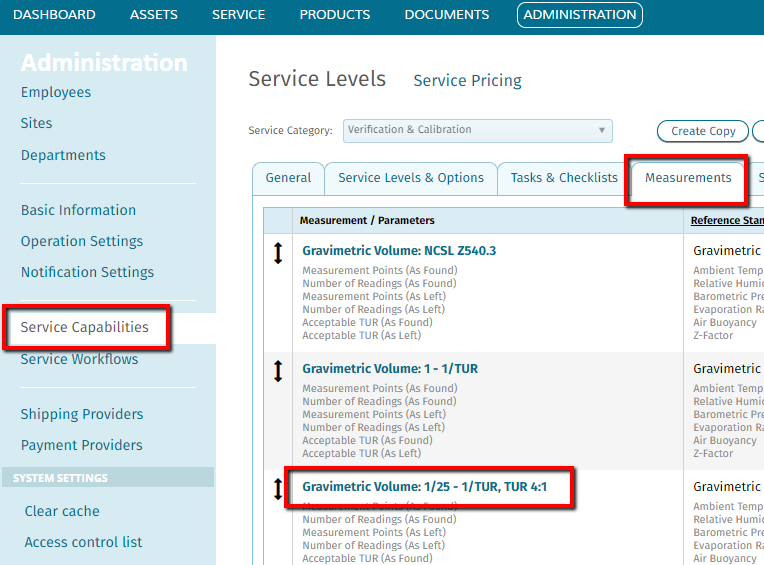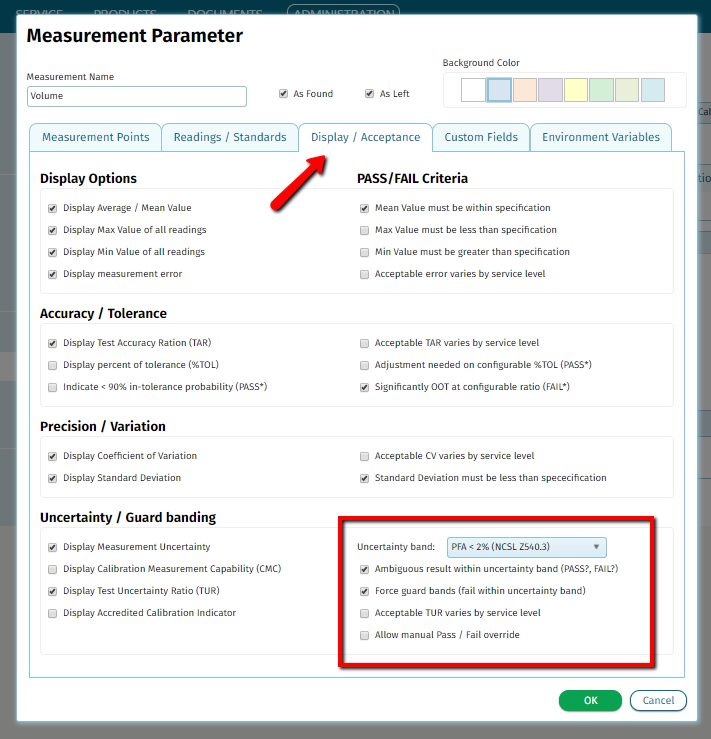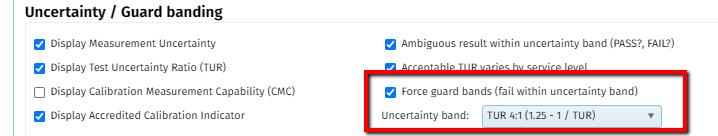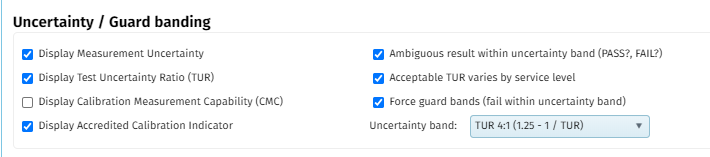# Overview

To set an uncertainty/guard band, go to the proper Measurement Parameter under the Measurements tab in the Service Capability.

Click ADMINISTRATION > Click "Service Capabilities" > Select x service level(s) to the right of the proper category > Click the "Measurements" tab > Click the Measurement/Parameter name:Click the "Display/Acceptance" tab.  In the bottom right corner will be all of the options and settings for uncertainty/guard bands.To access the "Uncertainty band:" drop down, select the "Force guard bands (fail within uncertainty band)" check box.Here you will see four formulas to assign to the "Uncertainty band" which all depend on the calculated Tool Uncertainty Ratio (TUR) value.

1. PFA (Probability of False Acceptance) < 2% (NCSL Z540.3) - uses the following formula for determining the widths of the guard band where:

• A is the acceptance limit
• T is the specification limit
• U is the measurement uncertainty (at the 95% confidence interval)
• M is the multiplier to calculate the guard band, determined empirically
• TUR is explained below in the TUR 4:1 section

A = T - U X M = T - U x 1.04 - e^(0.38log(TUR)-0.54)

2. Uncertainty (1 - 1 / TUR) where TUR is explained in the next step

3. TUR 4:1 (1.25 - 1 / TUR) - the goal is to have the standard being used on the unit under test (UUT) be 4x as more accurate than the UUT. If the TUR is 10:1, it will result in a pass since the standard is 10x more accurate. If the TUR is 0.5:1, it will result in a fail because the standard is not accurate enough.

TUR = (upper spec limit - lower spec limit) / 2 * expanded uncertainty

To set up the TUR in qualer, it may look like this:The ambiguous pass/fail is optional. When applied, the TUR in the data form may look like this:The TUR of 20:1 was achieved because (10.01 - 9.99) / 2 * 0.0005 = 20.

Please note: The guard bands are only applied when TUR is less than 4:1.

4. RDS (SQRT(1 - 1 / TUR^2)) where TUR was explained above

To set up TAR (Test Accuracy Ratio), visit this article: TAR Test Accuracy Ratio.